Question

# Determine the maturity date and compute interest for each note. (Use 360 days a year. Do...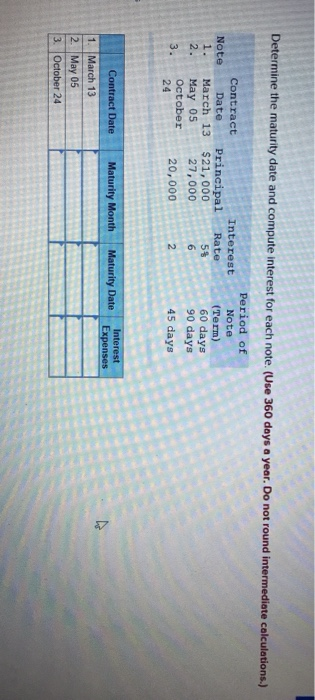Determine the maturity date and compute interest for each note. (Use 360 days a year. Do not round intermediate calculations.) Note 1. Interest Rate Contract Date Principal March 13 \$21,000 May 05 27,000 October 20,000 5\$ Period of Note (Term) 60 days 90 days 45 days 24 Maturity Month Maturity Date Interest Expenses Contract Date 1. March 13 2. May 05 3. October 24

Calculate following

 Contract Date Maturity month Maturity date Interest expenses 1 March 13 May 12 May 21000*5%*60/360 = 175 2 May 05 August 3 August 27000*6%*90/360 = 405 3 October 24 December 8 December 20000*2%*45/360 = 50

#### Earn Coins

Coins can be redeemed for fabulous gifts.

Similar Homework Help Questions
• ### Determine the maturity date and compute interest for each note. (Use 360 days a year. Do...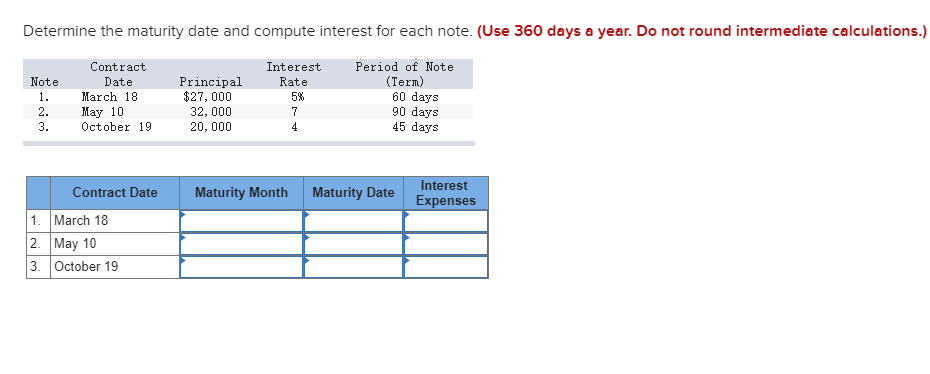Determine the maturity date and compute interest for each note. (Use 360 days a year. Do not round intermediate calculations.) Note Contract Date March 18 May 10 October 19 Interest Rate 5% 1. Period of Note (Term) 60 days 90 days 45 days Principal \$27,000 32,000 20,000 2. 3. Maturity Month Maturity Date Interest Expenses Contract Date 1. March 18 2. May 10 3. October 19

• ### Determine the maturity date and compute interest for each note. (Use 360 days a year. Do...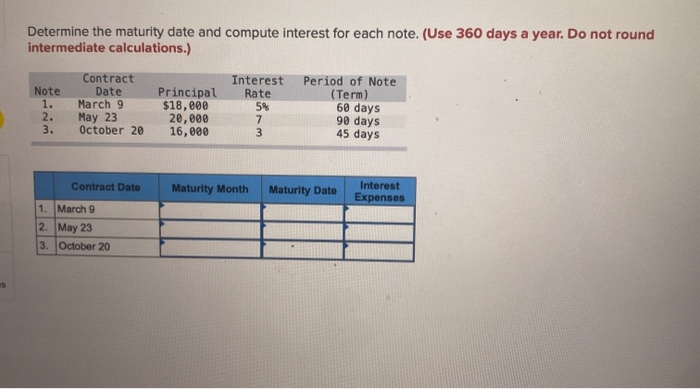Determine the maturity date and compute interest for each note. (Use 360 days a year. Do not round intermediate calculations.) Note 1. 2. 3. Contract Date March 9 May 23 October 20 Principal \$18,000 20,000 16,000 Interest Rate 5% 7 3 Period of Note (Term) 60 days 90 days 45 days Contract Date Maturity Month Maturity Dato Interest Expenses 1. March 9 2. May 23 3. October 20

• ### Determine the maturity date and compute interest for each note. (Use 360 days a year. Do...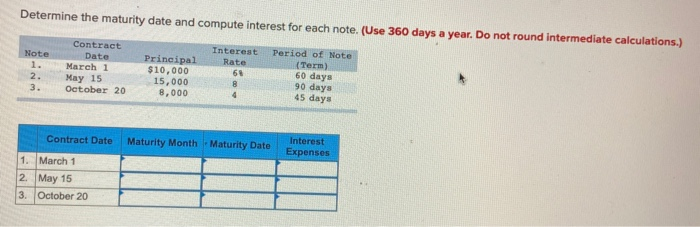Determine the maturity date and compute interest for each note. (Use 360 days a year. Do not round intermediate calculations.) Note Contract Date March 1 May 15 October 20 Principal \$10,000 15,000 8,000 Interest Period of Note Rate (Term 60 days 90 days 45 days Contract Date Maturity Month - Maturity Date Interest Expenses 1. March 1 2. May 15 3. October 20

• ### Determine the maturity date and compute interest for each note. (Use 360 days a year. Do...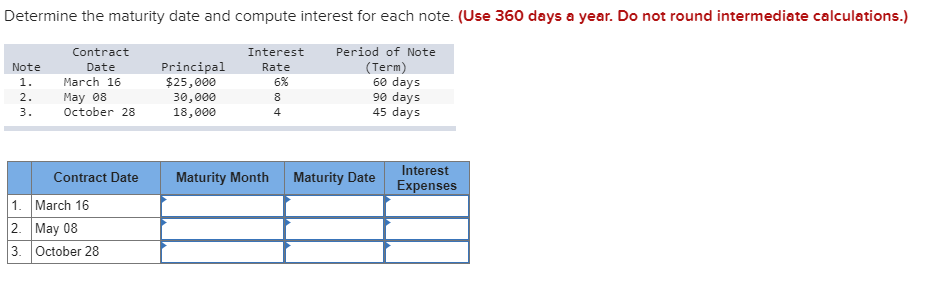Determine the maturity date and compute interest for each note. (Use 360 days a year. Do not round intermediate calculations.) Period of Note Contract Interest Principal \$25,000 (Term) 60 days 90 days 45 days Note Date Rate March 16 6% 1. May 08 October 28 2. 30,000 18,000 8 3. 4 Interest Maturity Month Maturity Date Contract Date Expenses 1. March 16 2. May 08 3 October 28

• ### 7 Homework Saved Determine the maturity date and compute interest for each note. (Use 360 days...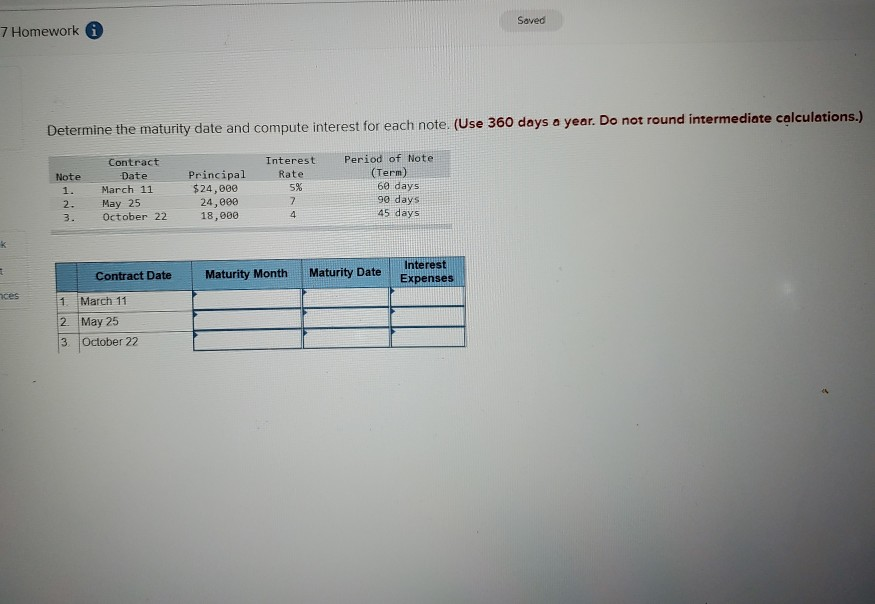7 Homework Saved Determine the maturity date and compute interest for each note. (Use 360 days a year. Do not round intermediate calculations.) Note Contract Date March 11 May 25 October 22 Interest Rate 5% Principal \$24,000 24,000 18,000 Period of Note (Term) 60 days 90 days 45 days 2. Contract Date Maturity Month Maturity Date Sate Interest Expenses ces 1 March 11 2 May 25 3 October 22

• ### Reload this page Naturtyates of Notes Receivable Determine the maturity date and compute the interest for...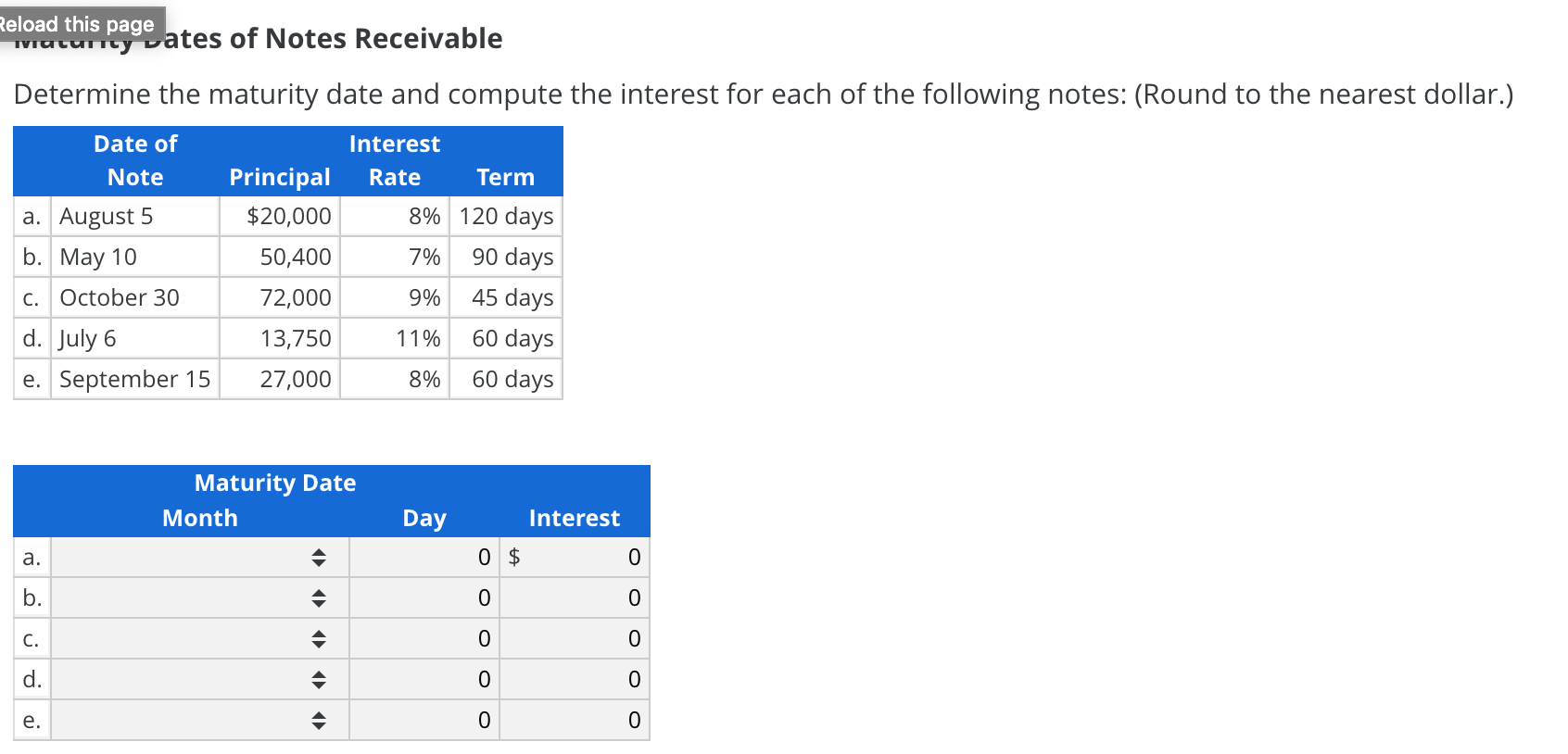Reload this page Naturtyates of Notes Receivable Determine the maturity date and compute the interest for each of the following notes: (Round to the nearest dollar.) Date of Note a. August 5 b. May 10 C. October 30 d. July 6 e. September 15 Principal \$20,000 50,400 Interest Rate 8% 7% 9% 11% 8% Term 120 days 90 days 45 days 60 days 60 days 72,000 13,750 27,000 Maturity Date Month Day Interest 0 \$ 0 ف ن ت نه

• ### Compute interest and find the maturity date for the following notes. (Use 360 days for calculation.)...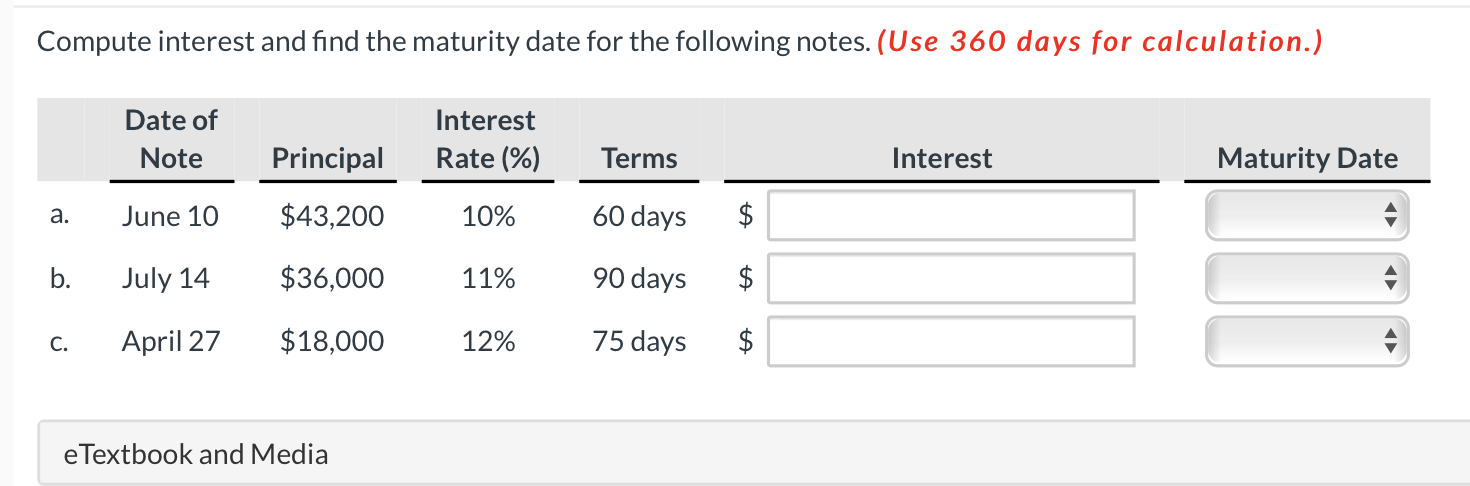Compute interest and find the maturity date for the following notes. (Use 360 days for calculation.) Date of Note Interest Rate (%) Principal Terms Interest Maturity Date a. June 10 \$43,200 10% 60 days \$ b. July 14 \$36,000 11% 90 days \$ C. April 27 \$18,000 12% 75 days \$ \$ e Textbook and Media

• ### Maturity Dates of Notes Receivable Determine the maturity date and compute the interest for each of...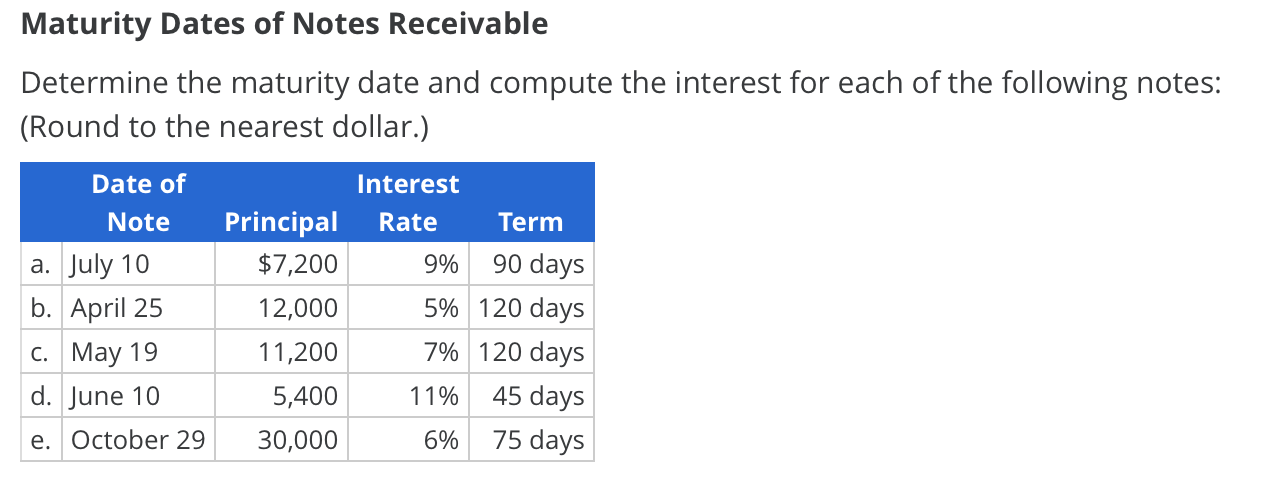Maturity Dates of Notes Receivable Determine the maturity date and compute the interest for each of the following notes: (Round to the nearest dollar.) Date of Interest Principal Rate Note Term 90 days \$7,200 а. July 10 9% 5% 120 days b. April 25 12,000 7% 120 days C. May 19 11,200 45 days d. June 10 5,400 11% 75 days e. October 29 30,000 6%

• ### Maturity Dates of Notes Receivable Determine the maturity date and compute the interest for each of...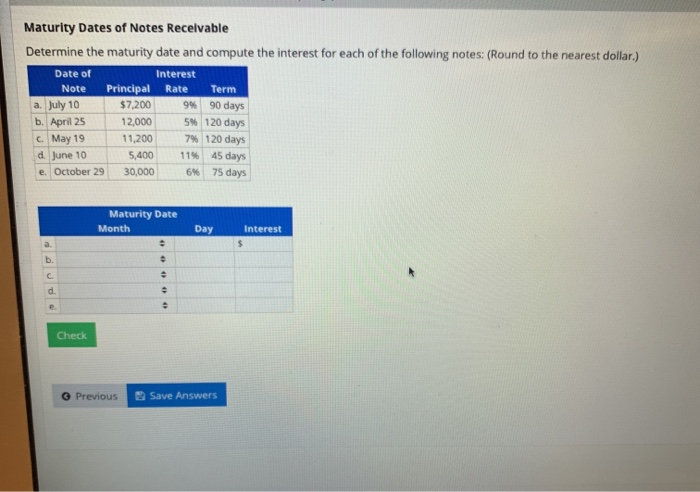Maturity Dates of Notes Receivable Determine the maturity date and compute the interest for each of the following notes: (Round to the nearest dollar.) Date of Interest Note Principal Rate Term a. July 10 \$7,200 94 90 days b. April 25 12,000 5 120 days May 19 11,200 7% 120 days d June 10 5.400 1145 days e. October 29 30,000 6% 75 days Maturity Date Month Day Interest Check Previous Save Answers

• ### Determine Due Date and Interest on Notes Determine the due date and the amount of interest due at maturity on the follo...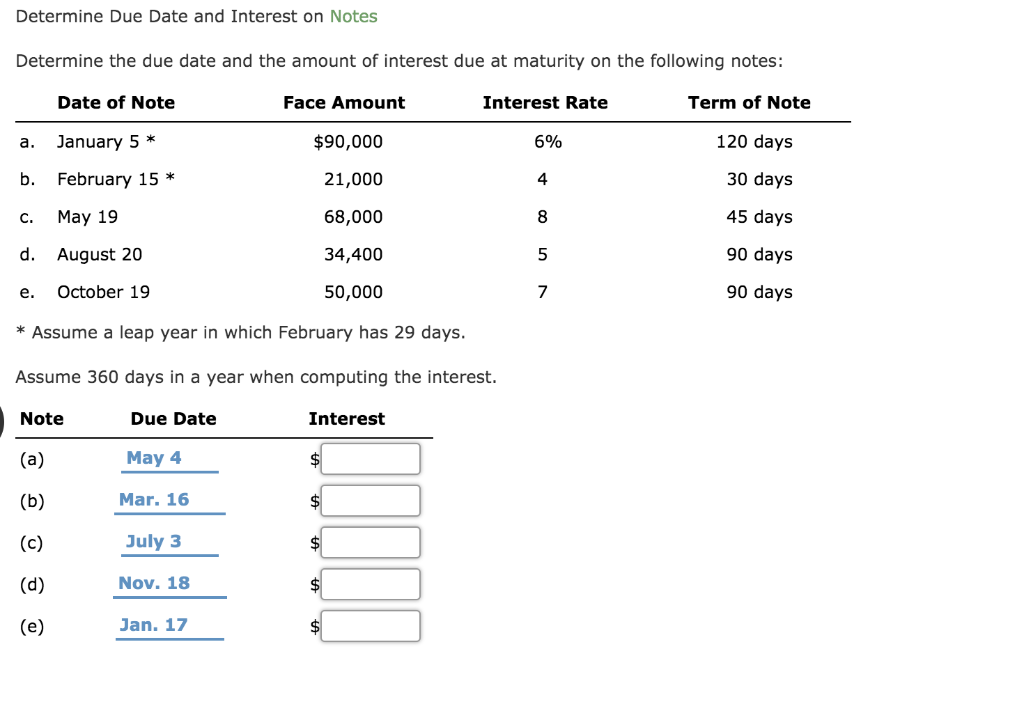Determine Due Date and Interest on Notes Determine the due date and the amount of interest due at maturity on the following notes: Date of Note Face Amount Interest Rate Term of Note \$90,000 120 days a. January 5* b. February 15 * C. May 19 30 days 21,000 68,000 45 days d. August 20 34,400 90 days e. October 19 50,000 90 days * Assume a leap year in which February has 29 days. Assume 360 days in a...